Open in App
Not now

# Class 12 RD Sharma Solutions – Chapter 30 Linear Programming – Exercise 30.3

• Last Updated : 20 May, 2021

### The minimum requirement of the nutrients in the diet is 1.00 mg of thiamine,7.50 mg of phosphorus, and 10.00 mg of iron. The cost of F1 is 20 paise per 25 grams while the cost of F2 is 15 paise per 25 grams. Find the minimum cost of the diet.

Solution:

Let 25x grams of food F1 and 25y grams of food F2 be used to fulfill the minimum requirement of thiamine, phosphorus and iron.

Given

The minimum requirement of the nutrients in the diet are 1.00 mg of thiamine,7.50 mg of phosphorus and 10.00 mg of iron. Therefore,

0.25x+0.10y ≥ 1

0.75x+1.50y ≥7.5

1.6x+0.8y ≥ 10

Since the quantity cannot be negative

⇒ x,y ≥ 0

The cost of F1 is 20 paise per 25 grams and the cost of F2 is 15 paise per 25 grams.

Therefore the cost of 25x grams of food F1 and 25y grams of food F2 is (0.20x+0.15y) rupees.

Let us minimize z=0.20x+0.15y

subject to

0.25x+0.10y ≥ 1

0.75x+1.50y ≥7.5

1.6x+0.8y ≥ 10

x, y ≥ 0

First we will convert the given inequations to equations. we get,

0.25x+0.10y = 1

0.75x+1.50y =7.5

1.6x+0.8y = 10

x=0 and y=0

Region represented by 0.25x+0.10y ≥ 1:

The line 0.25x+0.10y =1 meets the coordinate axis at A(4, 0) and B(0, 10).

Clearly (0, 0) do not satisfy the inequation 0.25x+0.10y≥1.So the region in XY plane that does not contain the origin represents the solution set of given inequation.

Region represented by 0.75x+1.50y ≥7.5:

The line 0.75x+1.50y =7.5 meets the coordinate axis at C(10, 0) and D(0, 5).

Clearly (0, 0) do not satisfy the inequation 0.75x+1.50y≥7.5.So the region in XY plane that doe snot contain the origin represents the solution set of this inequation.

Region represented by 1.6x+0.8y ≥ 10:

The line 1.6x+0.8y = 10 meets the coordinate axis at E(25/4, 0) and F(0, 25/2).

Clearly (0, 0) do not satisfy the inequation 1.6x+0.8y ≥ 10.So the region in XY plane that does not contain the origin represents the solution set of given inequation.

Region represented by x,y ≥ 0:

In the region represented by x≥0 and y≥0, every point in the first quadrant satisfies these inequations. So, the first quadrant is the region represented by these inequations.

The feasible region determined by the given constraints can be graphically represented as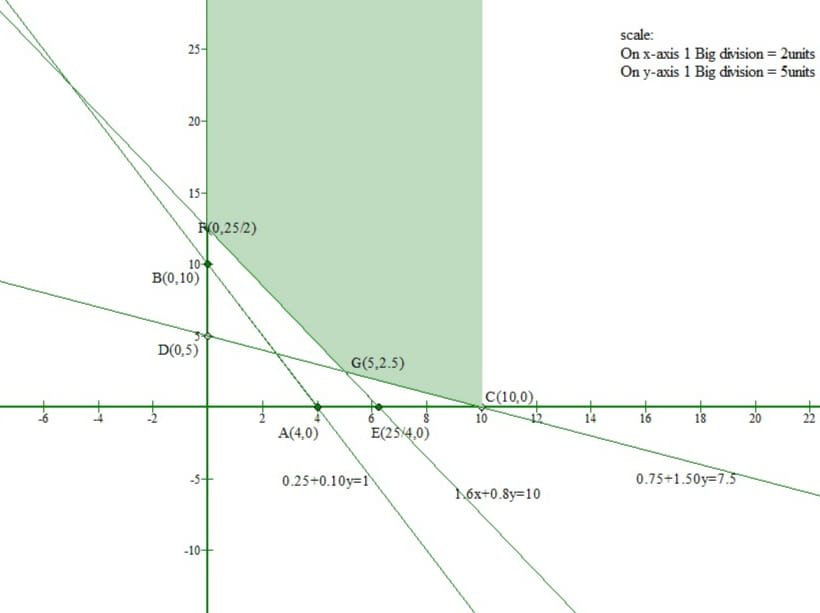The corner points of a Feasible region are F(0, 12.5), G(5, 2.5), C(10, 0)

The value of objective function at these points are given in the following table

Thus, the minimum cost is at G which is 1.375 rupees.

### Question 2. A diet for a sick person must contain at least 4000 units of vitamins, 50 units of minerals, and 1400 units of calories. Two foods A and B are available at a cost of Rs 4 and Rs 3 per unit respectively. If one unit of A contains 200 units of vitamin,1 unit of mineral and 40 calories and one unit of food B contains 100 units of vitamin, 2 units of minerals and 40 calories, find what combination of foods should be used to have the least cost?

Solution:

Let the sick person takes x units and y units of food A and B respectively.

1 unit of food A costs Rs 4 and that of food B costs Rs 3.

Therefore, x units of food A costs Rs 4x and y units of food B costs Rs 3y.

Total cost =Rs (4x+3y)

Let z=4x+3y

If one unit of A contains 200 units of vitamin and one unit of food B contains 100 units of vitamin.

Then, x units of food A and y units of food B contains (200x+100y) units of vitamin.

But a diet for a sick person must contain at least 4000 units of vitamin.

Thus 200x+100y≥4000

If one unit of A contains 1 unit of mineral and one unit of food B contains 2 units of mineral.

Then, x units of food A and y units of food B contains x+2y units of mineral.

But a diet for a sick person must contain at least 50 units of vitamin.

Thus, x+2y≥50

If one unit of A contains 40 calories and one unit of food B contains 40 calories.

Then x units of food A and y units of food B contains 40x+40y units of calories.

But a diet for a sick person must contain at least 1400 units of calories.

Thus, 40x+40y≥1400

Finally the quantities of food A and food B are nonnegative values.

So, x, y ≥ 0

Hence the required LPP is as follows:

Min z=4x+3y

subject to

200x+100y≥4000

x+2y≥50

40x+40y≥1400

x, y ≥ 0

First we will convert the given inequations to equations. we get,

200x+100y=4000

x+2y=50

40x+40y=1400

x=0 and y=0

Region represented by 200x+100y≥4000:

The line 200x+100y=4000 meets the coordinate axis at A1(20, 0) and B1(0, 40) respectively.

Clearly (0, 0) does not satisfy inequation 200x+100y≥4000.

So the region in XY plane that does not contain the origin represents the solution set of this inequation.

Region represented by x+2y≥50:

The line x+2y=50 meets the coordinate axis at C1(50, 0) and D1(0, 25) respectively.

Clearly (0, 0) does not satisfy inequation x+2y≥50.

So the region in XY plane that does not contain the origin represents the solution set of this inequation.

Region represented by 40x+40y≥1400:

The line 40x+40y=1400 meets the coordinate axis at E1(35, 0) and F1(0, 35) respectively.

Clearly (0, 0) does not satisfy inequation 40x+40y≥1400.

So the region in XY plane that does not contain the origin represents the solution set of this inequation.

Region represented by x≥ 0 and y≥0:

Every point in the first quadrant satisfies these inequations. So, the first quadrant is the region represented by these inequations.

The feasible region determined by the given constraints can be graphically represented as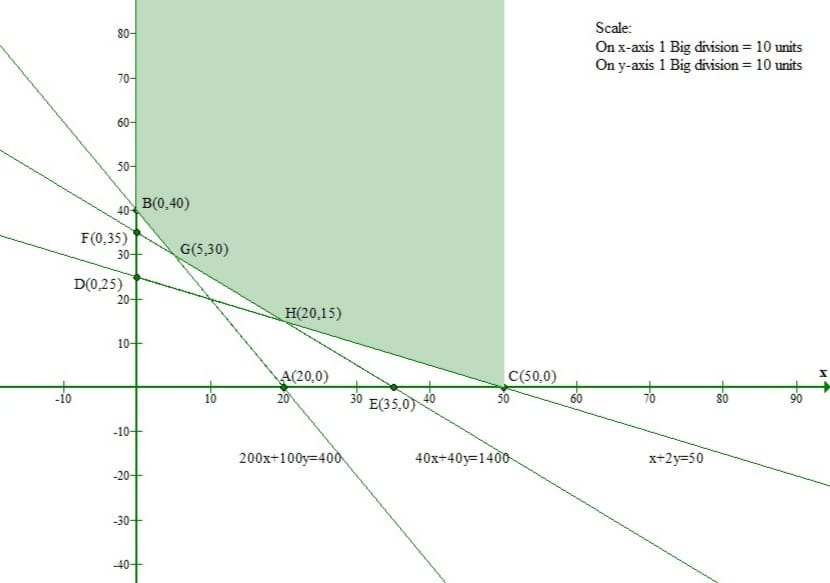The corner points of a Feasible region are B1(0, 40), G1(5, 30), C1(50, 0), H1(20, 15).

The value of objective function at these points are given in the following table

Thus the minimum cost is at G1 which is 110 rupees.

### Find the combination of food items so that the cost may be minimum.

Solution:

Let the person takes x units and y units of food I and II respectively.

Since, per unit of food I costs Rs 0.60 and that of food II costs Rs.1.00.

Therefore, x units of food I costs Rs 0.60x and y units of food II costs Rs1.00y.

Total cost per day=0.60x+1.00y

Let z=0.60x+1.00y

Since, each unit of food I contains 10 units of calcium.

Therefore, x units of food I contains 10x units of calcium.

Each unit of food II contains 4 units of Calcium.

So, y units of food II contains 4y units of calcium.

Thus, x units of food I and y units of food II contains (10x+4y) units of calcium.

But the minimum requirement is 20 units of calcium.

Thus, 10x+4y≥20

Since, each unit of food I contains 5 units of protein.

Therefore, x units of food I contains 5x units of protein.

Each unit of food II contains 6 units of protein.

So, y units of food II contains 6y units of protein.

Thus, x units of food I and y units of food II contains (5x+6y) units of protein.

But the minimum requirement is 20 units of protein.

Thus, 5x+6y≥20

Since, each unit of food I contains 2 units of Calories.

Therefore, x units of food I contains 2x units of Calories.

Each unit of food II contains 6 units of Calories.

So, y units of food II contains 6y units of Calories.

Thus, x units of food I and y units of food II contains (2x+6y) units of Calories.

But the minimum requirement is 12 units of Calories.

Thus, 2x+6y≥12

Finally the quantities of food I and food II are nonnegative values.

So, x, y ≥ 0

Hence the required LPP is as follows:

Min z=0.60x+1.00y

subject to

10x+4y≥20

5x+6y≥20

2x+6y≥12

x, y ≥ 0

First we will convert the given inequations to equations. we get,

10x+4y=20

5x+6y=20

2x+6y=12

x=0 and y=0

Region represented by 10x+4y≥20:

The line 10x+4y=20 meets the coordinate axes at A(2, 0) and B(0, 5) respectively.

Clearly (0, 0) does not satisfy inequation 10x+4y≥20.

So the region in XY plane that does not contain the origin represents the solution set of this inequation.

Region represented by 5x+6y≥20:

The line 5x+6y=20 meets the coordinate axes at C(4, 0) and D(0, 10/3) respectively.

Clearly (0, 0) does not satisfy inequation 5x+6y≥20.

So the region in XY plane that does not contain the origin represents the solution set of this inequation

Region represented by 2x+6y≥12:

The line 2x+6y=12 meets the coordinate axes at E(6, 0) and F(0, 2) respectively.

Clearly (0, 0) does not satisfy inequation 2x+6y≥12.

So the region in XY plane that does not contain the origin represents the solution set of this inequation.

Region represented by x, y ≥ 0:

Every point in the first quadrant satisfies these inequations. So, the first quadrant is the region represented by these inequations.

The feasible region determined by the given constraints can be graphically represented as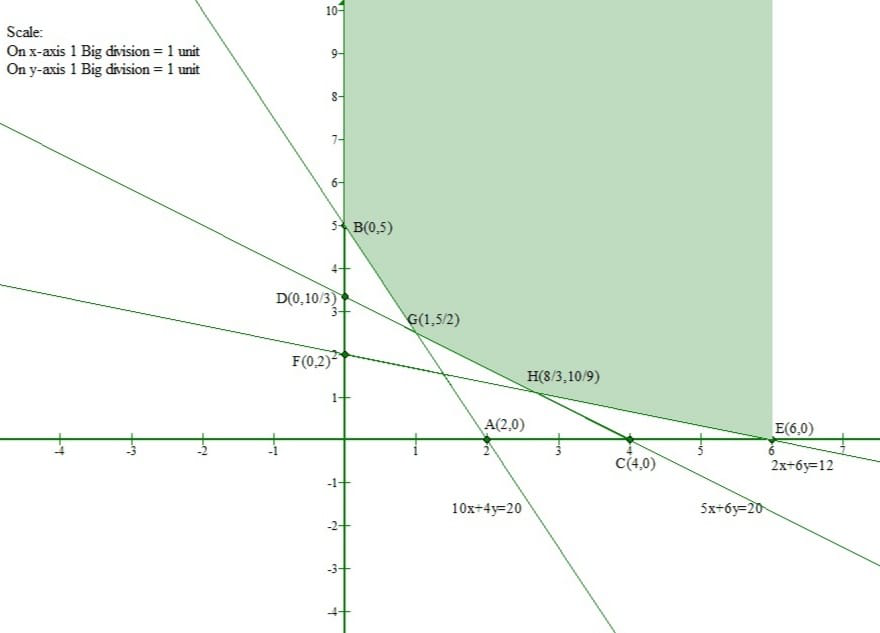The corner points of a Feasible region are B(0, 5), G(1, 5/2), E(6, 0), H(8/3, 10/9).

The value of objective function at these points are given in the following table

From the table, we can see that minimum cost is at H(8/3, 10/9) and that value is Rs 2.71

### Question 4. A hospital dietician wishes to find the cheapest combination of two foods, A and B that contains at least 0.5 milligrams of thiamine and at least 600 calories. Each unit of A contains 0.12 milligrams of thiamine and 100 calories, while each unit of B contains 0.10 milligrams of thiamin and 150 calories. If each food costs 10 paise per unit, how many units of each should be combined at a minimum cost?

Solution:

Let the dietician wishes to mix x units of food A and y units of food B

Therefore x,y≥0

The given information can be tabulated as follows

According to question,

The constraints are

0.2x+0.1y≥0.5

100x+150y≥600

It is given that each food costs 10 paise per unit

Let the total cost be z

z=10x+10y

Now the mathematical formulation of given linear programming problem is

0.2x+0.1y≥0.5

100x+150y≥600

Region represented by 0.12x+0.1y≥0.5:

The line 0.12x+0.1y=0.5 meets the coordinate axes at A1(25/6, 0) and B1(0, 5) respectively.

Clearly (0, 0) does not satisfy inequation 0.12x+0.1y≥0.5.

So the region in XY plane that does not contain the origin represents the solution set of this inequation.

Region represented by 100x+150y≥600:

The line 100x+150y=600 meets the coordinate axes at C1(6, 0) and D1(0, 4) respectively.

Clearly (0, 0) does not satisfy inequation 100x+150y≥600.

So the region in XY plane that does not contain the origin represents the solution set of this inequation.

Region represented by x,y≥0:

Every point in the first quadrant satisfies these inequations. So, the first quadrant is the region represented by these inequations.

The feasible region determined by the given constraints can be graphically represented as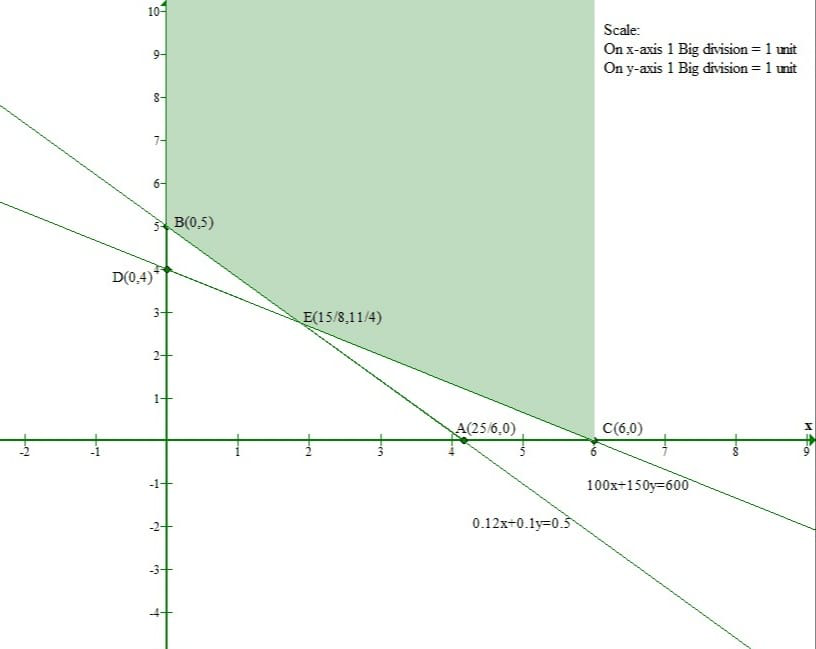The corner points of a Feasible region are B1(0, 5), E1(15/8, 11/4), C1(6, 0).

The value of objective function at these points are given in the following table

The minimum of z is at E1(15/8, 11/4) and the value is Rs 46.2.

Hence the cheapest combination of foods will be 1.875 units of food A and 2.75 units of food B.

### One kg of food X costs Rs 5 and one kg of Food Y costs Rs 8. Find the least cost of the mixture which will produce the desired diet.

Solution:

Let the dietician wishes to mix x kg of food X and y kg of food Y.

Therefore, x,y≥0

Given

It is given that the mixture should contain at least 6 units of vitamin A,7 units of vitamin B ,11 units of vitamin C and 9 units of vitamin D.

Therefore, the constraints are

x+2y≥6

x+y≥7

x+3y≥11

2x+y≥9

It is given that cost of food X is Rs 5 per kg and cost of food Y is Rs 8 per kg.

Let the total cost be z.

Thus, z=5x+8y

Now the mathematical formulation of given linear programming problem is

Minimize Z=5x+8y

subject to

x+2y≥6

x+y≥7

x+3y≥11

2x+y≥9

First we will convert the given inequations to equations. we get

x+2y=6

x + y=7

x+3y=11

2x+y=9

x=0 and y=0

Region represented by x+2y≥6:

The line x+2y=6 meets the coordinate axes at A1(6, 0) and B1(0, 3) respectively.

Clearly (0, 0) does not satisfy inequation x+2y≥6.

So the region in XY plane that does not contain the origin represents the solution set of this equation.

Region represented by x+y≥7:

The line x + y=7 meets the coordinate axes at C1(7, 0) and D1(0, 7) respectively.

Clearly (0, 0) does not satisfy inequation x+y≥7.

So the region in XY plane that does not contain the origin represents the solution set of this equation.

Region represented by x+3y≥11:

The line x+3y=11 meets the coordinate axes at E1(11, 0) and F1(0, 11/3) respectively.

Clearly (0, 0) does not satisfy inequation x+3y≥11.

So the region in XY plane that does not contain the origin represents the solution set of this equation.

Region represented by 2x+y≥9:

The line 2x+y=9 meets the coordinate axes at G1(9/2, 0) and H1(0, 9) respectively.

Clearly (0, 0) does not satisfy inequation 2x+y≥9.

So the region in XY plane that does not contain the origin represents the solution set of this equation.

Region represented by x,y≥0:

Every point in the first quadrant satisfies these inequations. So, the first quadrant is the region represented by these inequations.

The feasible region determined by the given constraints can be graphically represented as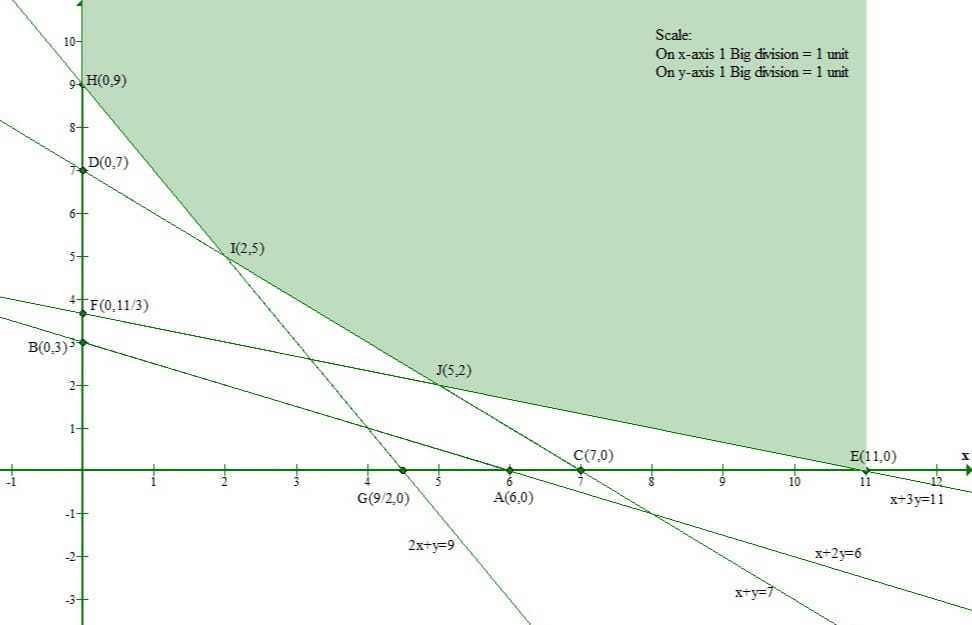The corner points of a Feasible region are H1(0, 9), I1(2, 5), E1(11, 0), J1(5, 2).

The value of objective function at these points are given in the following table.

The minimum value of z is at J1(5, 2) which is Rs 41.

Hence cheapest combination of foods will be 5 units of food X and 2 units of food Y.

### Question 6. A diet is to contain at least 80 units of vitamin A and 100 units of minerals. Two foods F1 and F2 are available.Food F1 costs Rs 4 per unit and F2 costs Rs 6 per unit. One unit of food F1 contains 3 units of vitamin A and 4 units of minerals.One unit of food F2 contains 6 units of vitamin A and 3 units of minerals. Formulate this as a linear programming problem and find graphically the minimum cost of diet that consists of mixture of these foods and also meets the mineral nutritional requirements.

Solution:

Let the dietician wishes to mix x units of food F1 and y units of food F2.

Clearly x,y≥0

The given information can be tabulated as follows

The constraints are

3x+6y≥80

4x+3y≥100

It is given that cost of food F1 is Rs 4 per unit and cost of food F2 is Rs 6 per unit.

Therefore cost of x units of food F1 and y units of food F2 is Rs 4x and Rs 6y respectively.

Let the total cost be z.

z=4x+6y

Now the mathematical formulation of given linear programming problem is

Minimize Z=4x+6y

subject to

3x+6y≥80

4x+3y≥100

x,y≥0

First we will convert the given inequations to equations. we get

3x+6y=80

4x+3y=100

x=0 and y=0

Region represented by 3x+6y≥80:

The line 3x+6y=80 meets the coordinate axes at A(80/3, 0) and B(0, 40/3) respectively.

Clearly (0, 0) does not satisfy inequation 3x+6y≥80.

So the region in XY plane that does not contain the origin represents the solution set of this equation.

Region represented by 4x+3y≥100:

The line 4x+3y=100 meets the coordinate axes at C(25, 0) and D(0, 100/3) respectively.

Clearly (0, 0) does not satisfy inequation 4x+3y≥100.

So the region in XY plane that does not contain the origin represents the solution set of this equation.

Region represented by x,y≥0:

Every point in the first quadrant satisfies these inequations. So, the first quadrant is the region represented by these inequations.

The feasible region determined by the given constraints can be graphically represented as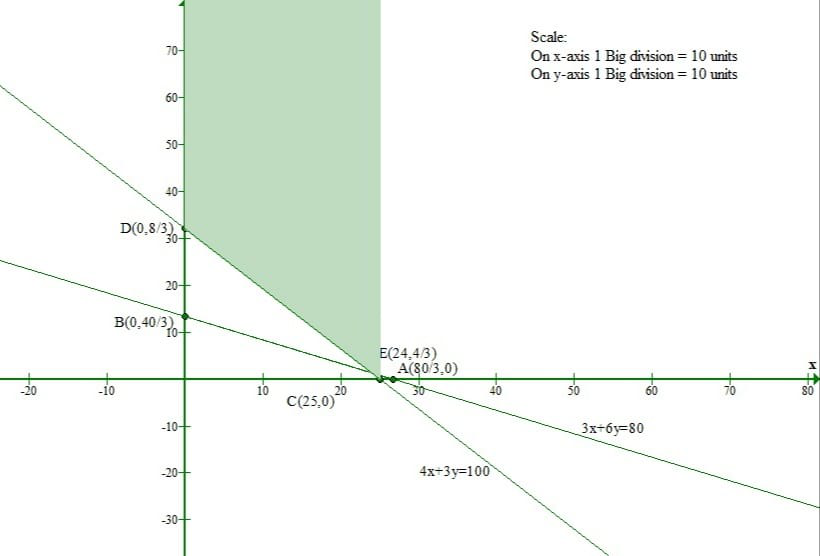The corner points of a Feasible region are D(0, 100/3), E(24, 4/3), A(80/3, 0).

The value of objective function at these points are given in the following table.

The minimum value of z is at E(24, 4/3) and the value is Rs 104.

### Question 7. Kellogg is a new cereal formed of a mixture of bran and rice that contains at least 88 grams of protein and at least 36 milligrams of iron. Knowing that bran contains 80 grams of protein and 40 milligrams of iron per kg and that rice contains 100 grams of protein and 30 milligrams of iron per kg, Find the minimum cost of producing this new cereal if bran costs Rs 5 per kg and rice costs Rs 4 per kg.

Solution:

Let the cereal contain x kg of bran and y kg of rice.

Therefore x,y≥0

The given information can be tabulated as follows

Bran and Rice contains at least 88 grams of protein and at least 36 milligrams of iron.

Thus the constraints are

80x+100y≥88

40x+30y≥36

It is given that bran costs Rs 5 per kg and rice costs Rs 4 per kg. Therefore cost of x kg of bran and y kg of rice is Rs 5x and Rs 4y respectively.

Hence, total profit is Rs(5x+4y).

Let the total cost be z

Thus, z=5x+4y

Now the mathematical formulation of given linear programming problem is

Minimize Z=5x+4y

subject to

80x+100y≥88

40x+30y≥36

x,y≥0

First we will convert the given inequations to equations. we get

80x+100y=88

40x+30y=36

x=0 and y=0

Region represented by 80x+100y≥88:

The line 80x+100y=88 meets the coordinate axes at A(1.1, 0) and B(0, 0.88) respectively.

Clearly (0, 0) does not satisfy inequation 80x+100y≥88.

So the region in XY plane that does not contain the origin represents the solution set of this equation.

Region represented by 40x+30y≥36:

The line 40x+30y=36 meets the coordinate axes at C(0.9, 0) and D(0, 1.2) respectively.

Clearly (0, 0) does not satisfy inequation 40x+30y≥36.

So the region in XY plane that does not contain the origin represents the solution set of this equation.

Region represented by x,y≥0:

Every point in the first quadrant satisfies these inequations. So, the first quadrant is the region represented by these inequations.

The feasible region determined by the given constraints can be graphically represented as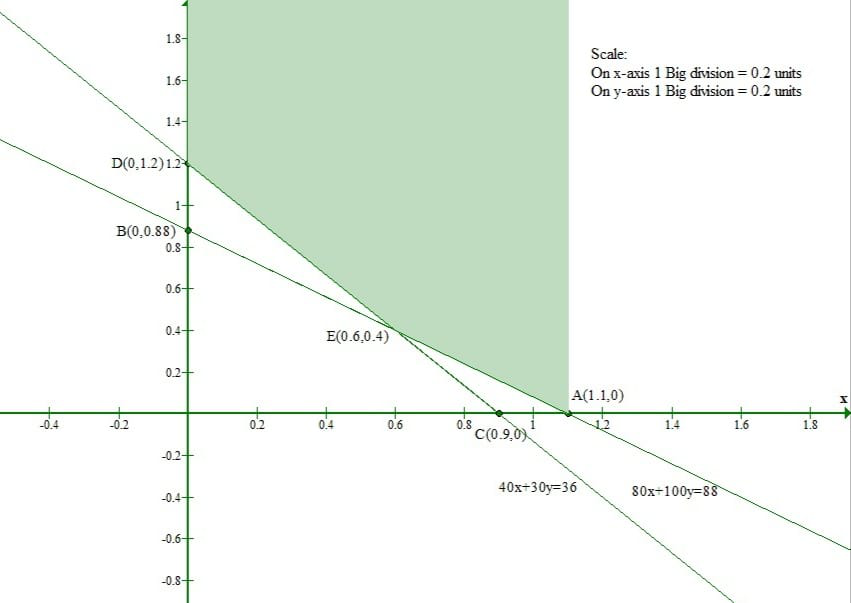The corner points of a Feasible region are D(0, 1.2), E(0.6, 0.4), A(1.1, 0).

The value of objective function at these points are given in the following table.

The minimum value of z is Rs 4.6 at E(0.6, 0.4)

### Question 8. A Wholesale dealer deals in two kinds A and B of the mixture of nuts. Each kg of mixture A contains 60 grams of almonds,30 grams of cashew nuts, and 30 grams of hazelnuts. Each kg of mixture B contains 30 grams of almonds,60 grams of cashew nuts, and 180 grams of hazelnuts. The remainder of both mixtures is per nuts. The dealer is contemplating using mixtures A and B to make a bag that will contain at least 240 grams of almonds,300 grams of cashew nuts,and 540 grams of hazelnuts. Mixture A costs Rs 8 per kg and mixture B costs Rs 12 per kg. Assuming that mixtures A and B are uniform, use the graphical method to determine the number of kg of each mixture which he should use to minimize the cost of the bag.

Solution:

Let x kg of kind A and y kg of kind B were used.

Since quantity cannot be negative.

Therefore x,y≥0

The given information can be tabulated as follows

Thus the constraints are

60x+30y≥240

30x+60y≥300

30x+180y≥540

Mixture A costs Rs 8 per kg and mixture B costs Rs 12 per kg

Total cost= 8x+12y

Let the total cost be z

Thus, z=8x+12y

Now the mathematical formulation of given linear programming problem is

Minimize Z=8x+12y

subject to

2x+y≥8

x+2y≥10

x+6y≥18

x,y≥0

First we will convert the given inequations to equations. we get

2x+y=8

x+2y=10

x+6y=18

x=0 and y=0

Region represented by 2x+y≥8:

The line 2x+y=8 meets the coordinate axes at A1(4, 0) and B1(0, 8) respectively.

Clearly (0, 0) does not satisfy inequation 2x+y≥8.

So the region in XY plane that does not contain the origin represents the solution set of this equation

Region represented by x+2y≥10:

The line x+2y=10 meets the coordinate axes at C1(10, 0) and D1(0, 5) respectively.

Clearly (0, 0) does not satisfy inequation x+2y≥10.

So the region in XY plane that does not contain the origin represents the solution set of this equation.

Region represented by x+6y≥18:

The line x+6y=18 meets the coordinate axes at E1(18, 0) and F1(0, 3) respectively.

Clearly (0, 0) does not satisfy inequation x+6y≥18.

So the region in XY plane that does not contains the origin represents the solution set of this equation

Region represented by x,y≥0:

Since every point in the first quadrant satisfies these inequations. So, the first quadrant is the region represented by these inequations.

The feasible region determined by the given constraints can be graphically represented as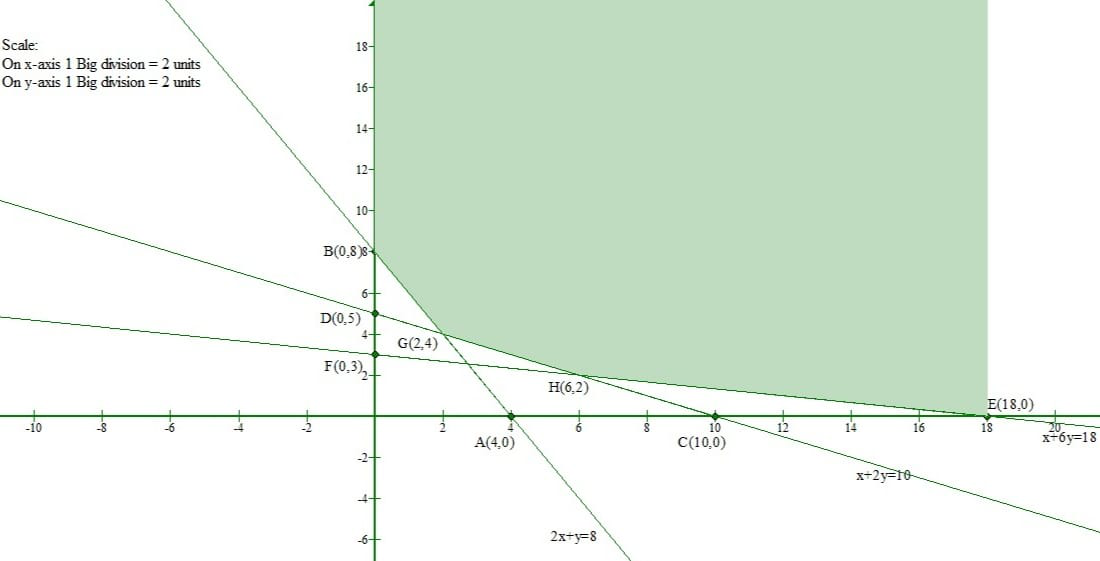The corner points of a Feasible region are B1(0, 8), G1(2, 4), H1(6, 2) and E1(18, 0).

The value of objective function at these points are given in the following table.

The minimum value of z is at G1(2, 4) and the value is Rs 64.

Thus the minimum cost is Rs 64 obtained when 2 units of kind A and 4 units of kind B nuts were used.

### Question 9. One kind of cake requires 300 grams of flour and 15 grams of fat, another kind of cake requires 150 gms of flour and 30 gms of fat. Find the maximum number of cakes that can be made from 7.5 kg of flour and 600 gms of fat, assuming that there is no shortage of other ingredients used in making the cake. Make it as an LPP and solve it graphically.

Solution:

Let there be x cakes of first kind and y cakes of second kind.

Therefore x,y≥0

The given information can be tabulated as follows

Thus the constraints are

300x+150y≤7500

15x+30y≤600

Total number of cakes that can be made =x + y

Let the total number of cakes be z.

Thus z=x + y

Now the mathematical formulation of given linear programming problem is

Maximize z=x + y

subject to

300x+150y≤7500

15x+30y≤600

x,y≥0

First we will convert the given inequations to equations. we get

300x+150y=7500

15x+30y=600

x=0 and y=0

Region represented by 300x+150y≤7500:

The line 300x+150y=7500 meets the coordinate axes at A(25, 0) and B(0, 50) respectively.

Clearly (0, 0) satisfies the equation 300x+150y=7500.

So the region which contains origin that represents the solution set of this inequation.

Region represented by 15x+30y≤600:

The line 15x+30y=600 meets the coordinate axes at C(40, 0) and D(0, 20) respectively.

Clearly (0, 0) satisfies the equation 15x+30y=600.

So the region in XY plane that contains the origin represents the solution set of this inequation.

Region represented by x,y≥0:

Since every point in the first quadrant satisfies these inequations. So, the first quadrant is the region represented by these inequations.

The feasible region determined by the given constraints can be graphically represented as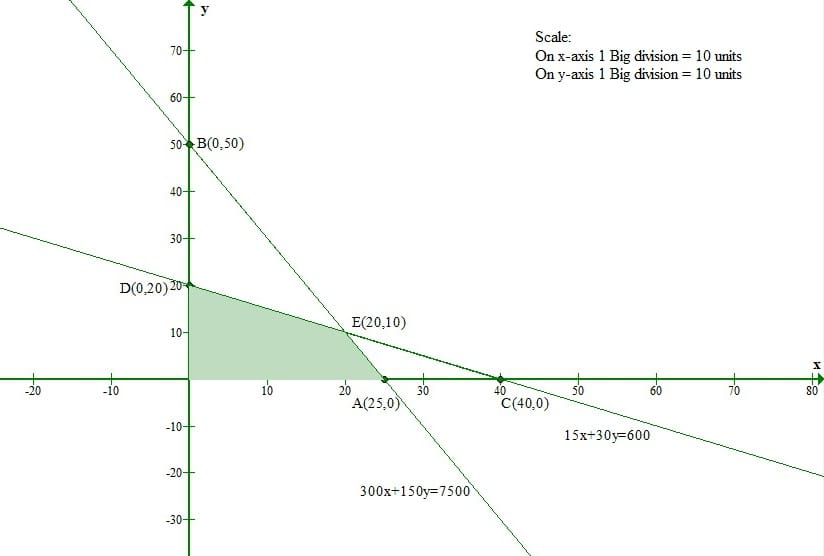The corner points of a Feasible region are O(0, 0), A(25, 0), D(0, 20) and E(20,10).

The value of objective function at these points are given in the following table.

Thus maximum number of cakes is 30 and that can be made from 20 of the first kind and 10 of other kind.

### Question 10. Reshma wishes to mix two types of food P and Q in such a way that the vitamin contents of the mixture contain at least 8 units of vitamin A and 11 units of vitamin B. Food P costs Rs 60 per kg and food Q costs Rs 80 per kg. Food P contains 3 units per kg of vitamin A and 5 units per kg of vitamin B while food Q contains 4 units per kg of vitamin A and 2 units per kg of vitamin B. Determine the minimum cost of the mixture.

Solution:

Let x units of food P and y units of food Q are mixed together to make the mixture.

The cost of food P is Rs 60 per kg and that of food Q is Rs 80 per kg.

So, x kg of food P and y kg of food Q will cost Rs(60x+80y).

Since one kg of food P contains 3 units of vitamin A and one kg of food Q contains 4 units of vitamin A, therefore x kg of food P and y kg of food Q will contain (3x+4y) units of vitamin A. But the mixture should contain at least 8 units of vitamin A.

Thus, 3x+4y≥8

Similarly x kg of food P and y kg of food Q will contain 5x+2y units of vitamin B. But the mixture should contain at least 11 units of vitamin B.

Thus, 5x+2y≥11

Now the mathematical formulation of given linear programming problem is

Minimize z=60x+80y

subject to

3x+4y≥8

5x+2y≥11

x,y≥0

The feasible region determined by the given constraints can be graphically represented as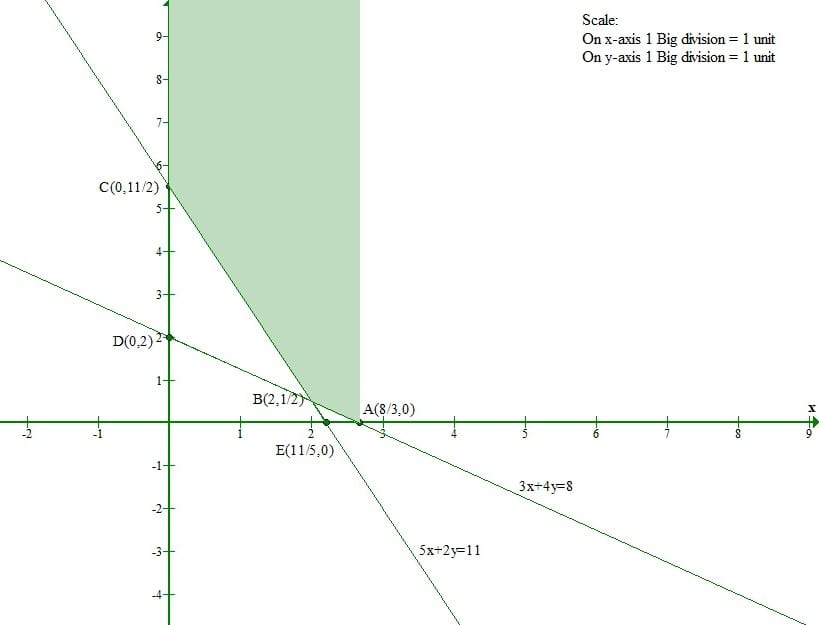The corner points of a Feasible region are A(8/3, 0), B(2, 1/2), C(0, 11/2).

The value of objective function at these points are given in the following table.

The smallest value of z is 160 at A(8/3, 0) and B(2, 1/2).

It can be verified that the open half-plane represented by 60x+80y<160 has no common points with the feasible region.

So, the minimum cost of mixture is Rs 160 since the minimum value of z is 160.

### Question 11. One kind of cake requires 200g of flour and 25 gms of fat and another kind of cake requires 100 gms of flour and 50 grams of fat. Find the maximum number of cakes that can be made from 5 kg of flour and 1 kg of fat. Assuming that there is no storage of the other ingredients used in making the cakes.

Solution:

Let the number of cakes of one kind and another kind be x and y respectively.

Therefore, total number of cakes produced are (x+y).

One kind of cake requires 200g of flour and another kind of cake requires 100 gms of flour.

So, x cakes of one kind and y cakes of another kind requires (200x+100y) grams of flour. But cakes should contain at most 5 kg of flour.

Thus, 200x+100y≤5000

⇒  2x+y≤50

One kind of cake requires 25g of fat and another kind of cake requires 50 gms of fat.

So, x cakes of one kind and y cakes of another kind requires (25x+50y) grams of fat. But cakes should contain at most 1 kg of fat.

Thus, 25x+50y≤1000

⇒ x+2y≤40

Now the mathematical formulation of given linear programming problem is

Minimize z=x + y

subject to

2x+y≤50

x+2y≤40

x,y≥0

The feasible region determined by the given constraints can be graphically represented as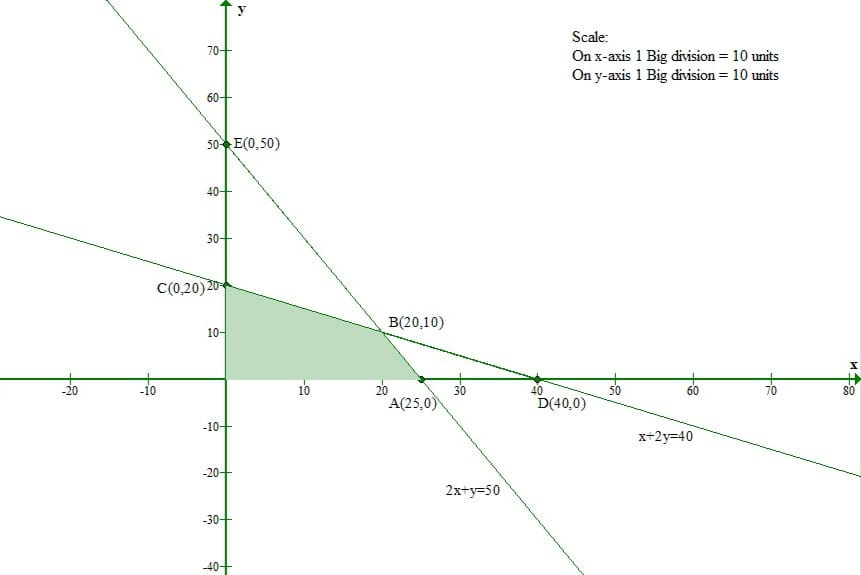The corner points of a Feasible region are O(0, 0),  A(25, 0), B(20, 10), C(0, 20).

The value of objective function at these points are given in the following table.

Thus the maximum value of z is 30 at B(20,10).

Hence the maximum number of cakes which can be made are 30.

### Question 12. A dietician has to develop a special diet using two foods P and Q. Each packet (containing 30 grams) of food P contains 12 units of calcium, 4 units of iron, 6 units of cholesterol and 6 units of vitamin A. Each packet of same quantity of food Q contains 3 units of calcium, 20 units of iron, 4 units of cholesterol and 3 units of vitamin A. The diet requires at least 240 units of calcium, at least 460 units of iron and atmost 300 units of cholesterol. How many packets of each food should be used to minimize the amount of vitamin A in the diet? What is the minimum of vitamin A?

Solution:

Let x packets of food P and y packets of food Q be used to make the diet.

Each packet of food P contains 6 units of vitamin A and each packet of food Q contains 3 units of vitamin A.

Therefore, x packets of food P and y packets of food Q contains (6x+3y) units of vitamin A.

Since each packet of food P contains 12 units of calcium and each packet of food Q contains 3 units of calcium.

Therefore, x packets of food P and y packets of food Q will contain (12x+3y) units of calcium. But the diet should contain at least 240 units of calcium.

Thus 12x+3y≥240

⇒ 4x+y≥80

Similarly,  x packets of food P and y packets of food Q contains (4x+20y) units of iron.

But the diet should contain at least 460 units of iron.

Thus 4x+20y≥460

⇒ x+5y≥115

Also, x packets of food P and y packets of food Q contains (6x+4y) units of cholesterol.

But the diet should contain atmost 300 units of cholesterol.

Thus 6x+4y≤300

⇒ 3x+2y≤150

Now the mathematical formulation of given linear programming problem is

Minimize z=6x+3y

subject to

4x+y≥80

x+5y≥115

3x+2y≤150

x,y≥0

The feasible region determined by the given constraints can be graphically represented as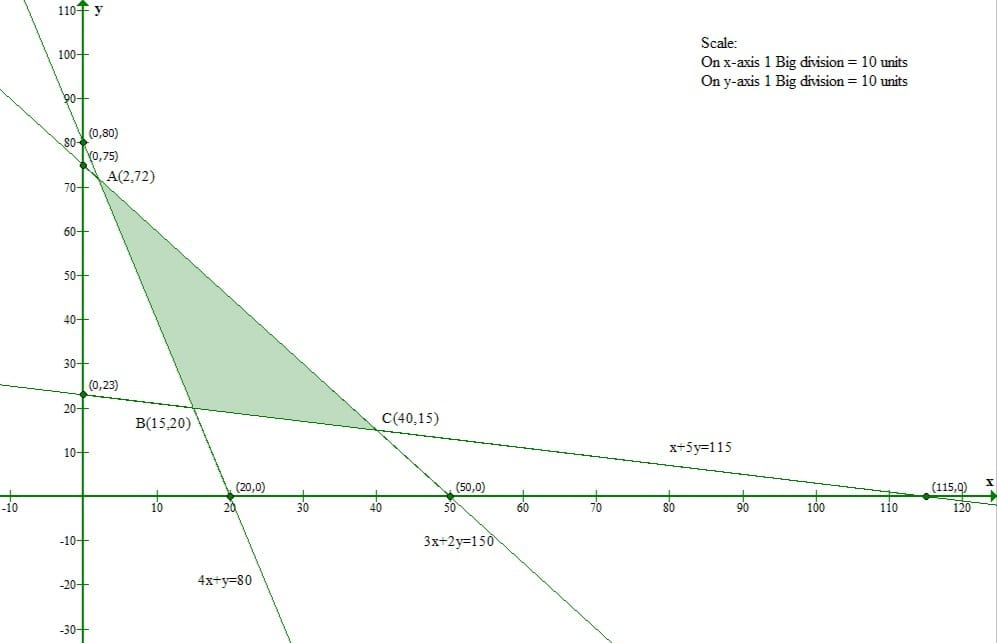The corner points of a Feasible region are A(2, 72), B(15, 20), C(40, 15).

The value of objective function at these points are given in the following table.

The smallest value of z is 150 which is obtained at point B(15, 20).

Thus,15 packets of food P and 20 packets of food Q should be used to minimize the amount of vitamin A on the diet.

Hence, the minimum amount of vitamin A is 150 units.

### Question 13. A farmer mixes two brands of P and Q of cattle feed.Brand P, costing Rs 250 per bag, contains 3 units of nutritional element A, 2.5 units of element B and 2 units of element C. Brand Q costing Rs 200 per bag contains 1.5 units of nutritional element A, 11.25 units of element B and 3 units of element C. The minimum requirements of nutrients A, B and C are 18 units,45 units and 24 units respectively. Determine the number of bags of each brand which should be mixed in order to produce a mixture having a cost per bag? What is the minimum cost of mixture per bag?

Solution:

Let x bags of brand P and y bags of brand Q should be mixed to produce the mixture.

Each bag of brand P costs Rs 250 per bag and each bag of brand Q costs Rs 200 per bag.

Therefore, x bags of brand P and y bags of brand Q costs Rs(250x+200y).

Since each bag of brand P contains 3 units of nutritional element A and each bag of brand Q contains 1.5 units of nutritional element A.

Therefore, x bags of brand P and y bags of brand Q will contain (3x+1.5y) units of nutritional element A.

But the minimum requirement of nutrients A is 18 units.

Thus, 3x+1.5y≥18

⇒  2x+y≥12

Similarly, x bags of brand P and y bags of brand Q will contain (2.5x+11.25y) units of nutritional element B.

But the minimum requirement of nutrients B is 45 units.

Thus, 2.5x+11.25y≥45

⇒  2x+9y≥36

Also, x bags of brand P and y bags of brand Q will contain (2x+3y) units of nutritional element C.

But the minimum requirement of nutrients C is 24 units.

Thus, 2x+3y≥24

Now the mathematical formulation of given linear programming problem is

Minimize z=250x+200y

subject to

2x+y≥12

2x+9y≥36

2x+3y≥24

x,y≥0

The feasible region determined by the given constraints can be graphically represented as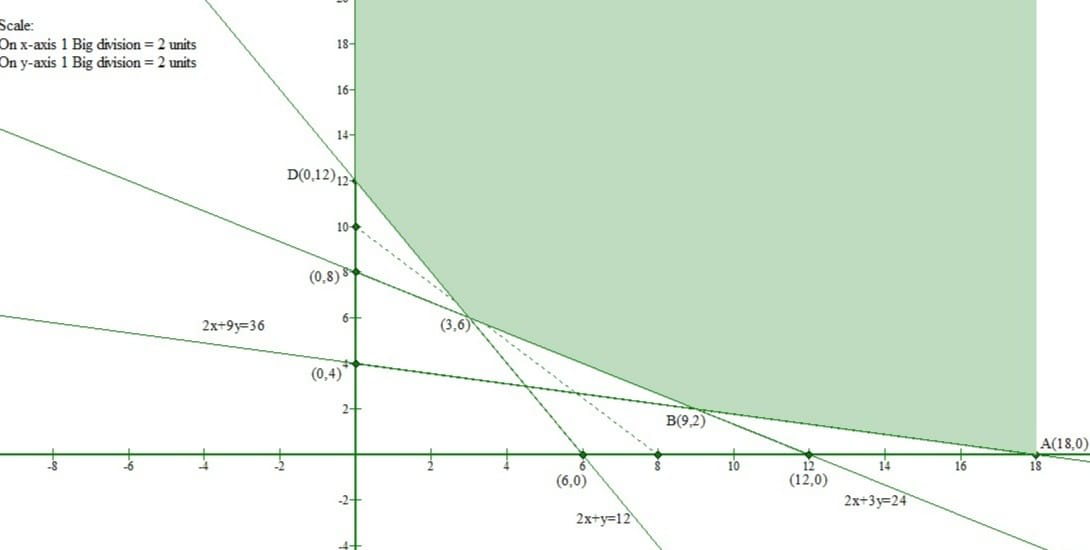The corner points of a Feasible region are A(18, 0), B(9, 2), C(3, 6), D(0, 12).

The value of objective function at these points are given in the following table.

The smallest value of z is 1950 obtained at C(3, 6).

So, 3 bags of brand P and 6 bags of brand Q should be used to minimize the cost.

Hence, the minimum cost of mixture per bag is Rs 1950.

### One kg of food X costs Rs 16 and one kg of food Y costs Rs 20. Find the least cost of mixture which will produce the required diet?

Solution:

Let x kg of food X and y kg of food Y are mixed together to make the mixture.

The cost of food X is Rs 16 per kg and that of food Y is Rs 20 per kg.

So, x kg of food X and y kg of food Y will cost Rs (16x+20y).

Since one kg of food X contains 1 unit of vitamin A and one kg of food Y contains 2 units of vitamin A. Therefore, x kg of food X and y kg of food Y will contain (x+2y) units of vitamin A.

But the mixture should contain at least 10 units of vitamin A.

Thus, x+2y≥10

Similarly x kg of food X and y kg of food Y will contain (2x+2y) units of vitamin B. But the mixture should contain at least 12 units of vitamin B.

Thus, 2x+2y≥12

⇒ x+y≥6

Also, x kg of food X and y kg of food Y will contain (3x+y) units of vitamin C. But the mixture should contain at least 8 units of vitamin C.

Thus, 3x+y≥8

Now the mathematical formulation of given linear programming problem is

Minimize z=16x+20y

subject to

x+2y≥10

x+y≥6

3x+y≥8

x,y≥0

The feasible region determined by the given constraints can be graphically represented as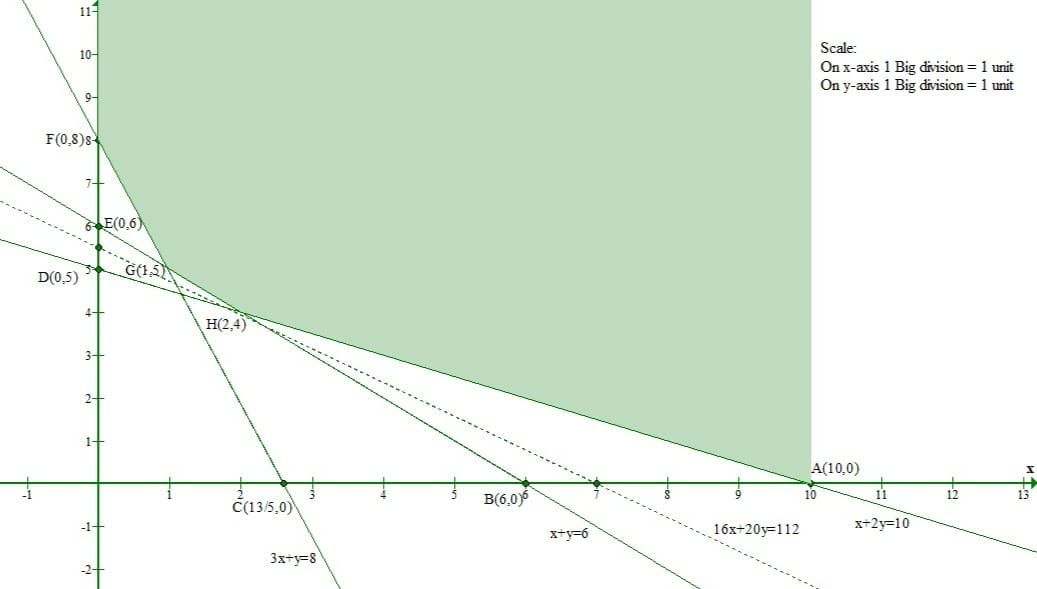The corner points of a Feasible region are A(10, 0), H(2, 4), G(1, 5), F(0,8).

The value of objective function at these points are given in the following table.

The smallest value of z is 112 obtained at H(2, 4).

Hence the least cost of mixture which will produce the required diet is Rs 112.

### If the grower wants to minimize the amount of nitrogen added to the garden, how many bags of each brand should be used? What is the minimum amount of nitrogen added to the garden?

Solution:

Let x bags of fertilizer of brand P and y bags of fertilizer of brand Q are used in the garden.

One bag of brand P contains 3 kg of nitrogen and one bag of brand Q contains 3.5 kg of nitrogen, therefore, x bags of brand P and y bags of brand Q will contain (3x+3.5y) kg of nitrogen.

Since, One bag of brand P contains 1 kg of phosphoric acid and one bag of brand Q contains 2 kg of phosphoric acid, therefore, x bags of brand P and y bags of brand Q will contain (x+2y) kg of phosphoric acid.

But the garden needs at least 240 kg of phosphoric acid.

Thus, x+2y≥240

Similarly, One bag of brand P contains 3 kg of potash and one bag of brand Q contains 1.5 kg of potash, therefore, x bags of brand P and y bags of brand Q will contain (3x+1.5y) kg of potash.

But the garden needs at least 270 kg of  potash.

Thus, 3x+1.5y≥270

⇒  2x+y≥180

Also, One bag of brand P contains 1.5 kg of Chlorine and one bag of brand Q contains 2 kg of Chlorine, therefore, x bags of brand P and y bags of brand Q will contain (1.5x+2y) kg of Chlorine.

But the garden needs atleast 310 kg of  Chlorine.

Thus, 1.5x+2y≤310

Now the mathematical formulation of given linear programming problem is

Minimize z=3x+3.5y

subject to

x+2y≥240

2x+y≥180

1.5x+2y≤310

x,y≥0

The feasible region determined by the given constraints can be graphically represented as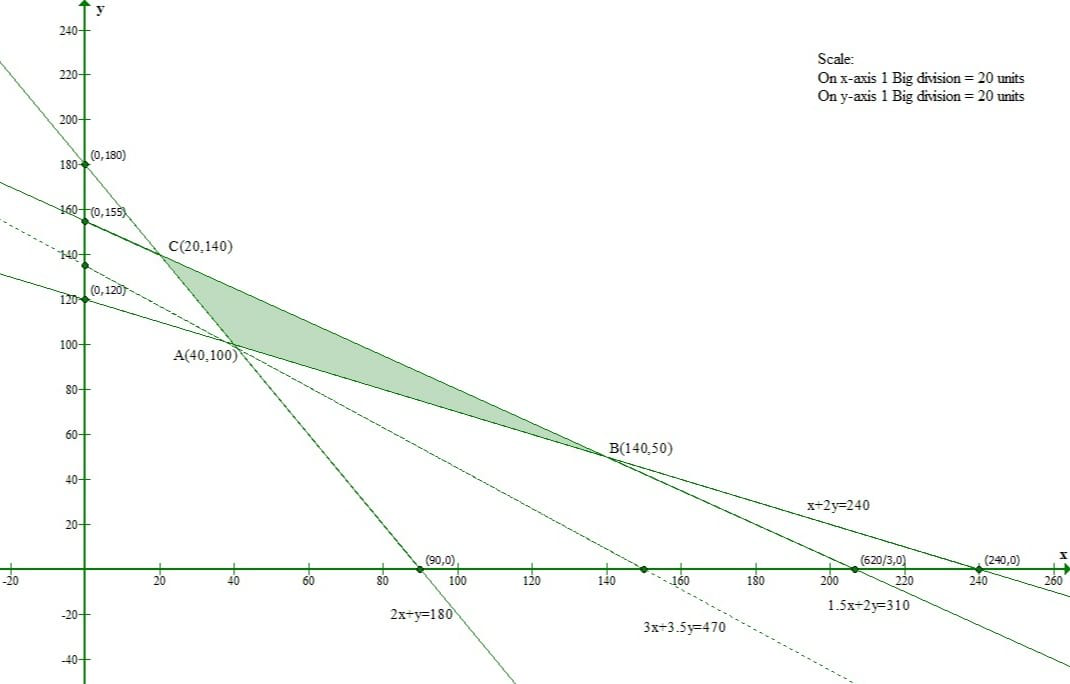The corner points of a Feasible region are A(40, 100), B(140, 50), C(20, 140).

The value of objective function at these points are given in the following table.

The smallest value of z is 470 obtained at A(40, 100).

Hence 40 bags of fertilizer of brand P and 100 bags of fertilizer of brand Q are used in the garden. The minimum amount of nitrogen added in the garden is 470 kg.

My Personal Notes arrow_drop_up
Related Articles